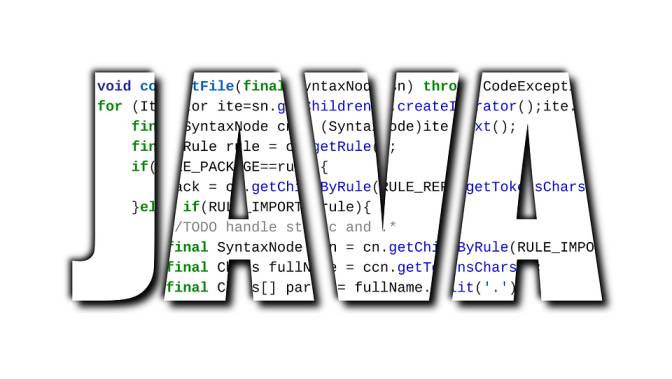# References and Primitive Data TypesTwo types of entities are identified by JAVA

• Primitive
• Objects

## Primitive Data Types in JAVA

• most basic types of data
• 8 primitive data types
• They have fixed size
• They are limited to range of value
• byte
• has size of 8 bits
• minimum value is -128
• maximum value is +127
• example : byte c=32;
• short
• size of 16 bits
• minimum value: -2^15
• maximum value: (2^15)-1
• example: short s=55;
• char
• size of 16 bits
• minimum value: 0
• maximum value: (2^16)-1
• All Unicode characters are char
• int
• size: 32 bits
• minimum value: 0-2^31
• maximum value: (2^31_-1
• Example: int i=25;
• long
• 64 bits size
• minimum value: (-2)^31
• maximum value: (2^31)-1
• Float
• Size: 32 bits
• minimum value: 2^-149
• maximum value: (2-2^-23)·2^127
• double
• 64 bits size
• +/-10-308 to +/-10+308 and 0, about 15 digits precision
• boolean
• True or false

## Reference variables

store the address of an object

No notion of “object (physically) in the stack”

No notion of “object (physically) within an object